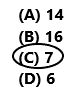# Texas Go Math Grade 5 Lesson 6.3 Answer Key Fraction and Whole-Number Multiplication

Refer to our Texas Go Math Grade 5 Answer Key Pdf to score good marks in the exams. Test yourself by practicing the problems from Texas Go Math Grade 5 Lesson 6.3 Answer Key Fraction and Whole-Number Multiplication.

## Texas Go Math Grade 5 Lesson 6.3 Answer Key Fraction and Whole-Number Multiplication

Unlock the Problem

Charlene has five 1-pound bags of different color sands. For an art project, she will use $$\frac{3}{8}$$ pound of each bag of sand to create a colorful sand-art jar. How much sand will be in Charlene’s sand-art jar?

• How much sand is in each bag?
____________________
• Will Charlene use all of the sand in each bag? Explain.
____________________

Multiply a fraction by a whole number.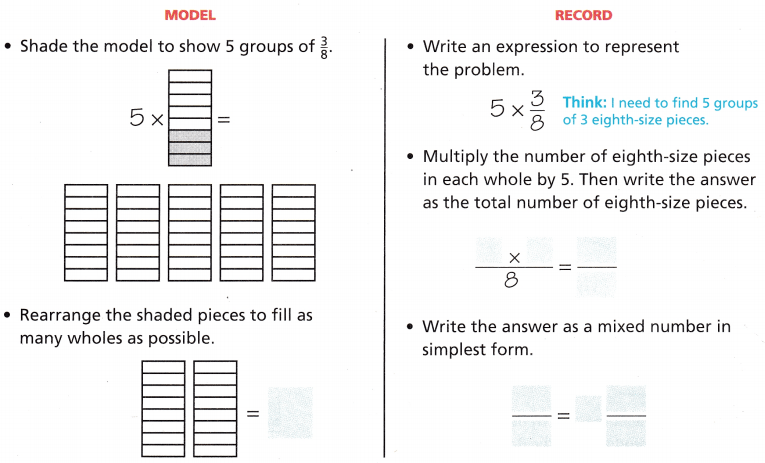So, there are _________ pounds of sand in Charlene’s sand -art jar.

• How much sand is in each bag?
1 pound
• Will Charlene use all of the sand in each bag? Explain.
No, she will use 3/8 pound of the sand in each bag.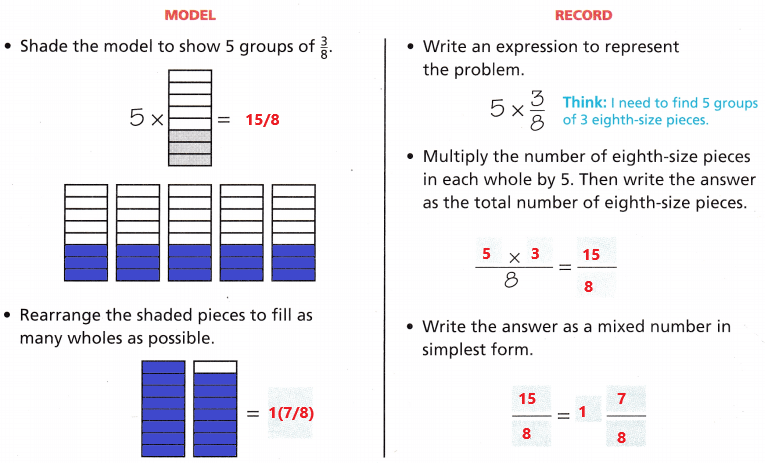So, there are 1(7/8) pounds of sand in Charlene’s sand -art jar.
Math Talk

Mathematical Processes

Explain how you can find how much sand Charlene has left.

Example

Multiply a whole number by a fraction.
Kirsten brought in 4 loaves of bread to make sandwiches for the class picnic. Her classmates used $$\frac{2}{3}$$ of the bread. How many loaves of bread were used?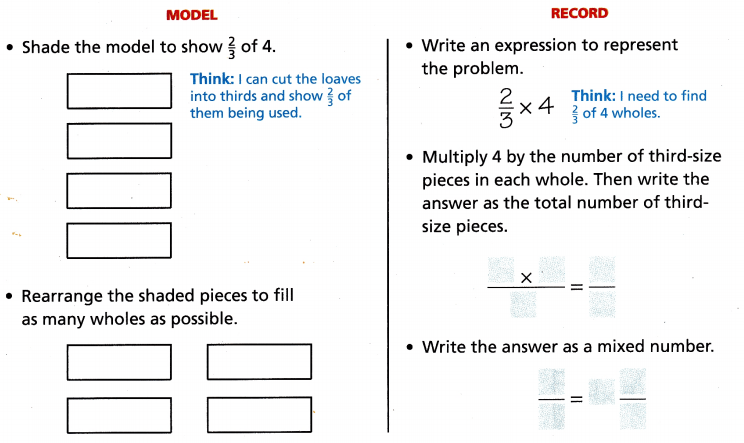So, ________ loaves of bread were used.So, 2(2/3) loaves of bread were used.

Share and Show

Find the product. Write the product in simplest form.

Question 1.
3 × $$\frac{2}{5}$$ = ___________• Multiply the numerator by the whole number. Write the product over the denominator.
• Write the answer as a mixed number in simplest form.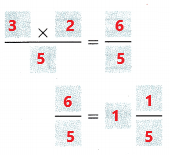Explanation:
In the above image we can observe the expression 3 x (2/5). First multiply the two whole numbers in the numerator. Multiply 3 with 2 the product is 6. Write the product 6 over the denominator 5. Write the fraction 6/5 in simplest form as a mixed number. The mixed number is 1(1/5).

Question 2.
$$\frac{2}{3}$$ × 5 = ___________Explanation:
In the above image we can observe the expression (2/3) x 5. First multiply the two whole numbers in the numerator. Multiply 2 with 5 the product is 10. Write the product 10 over the denominator 3. Write the fraction 10/3 in simplest form as a mixed number. The mixed number is 3(1/3).

Question 3.
6 × $$\frac{2}{3}$$ = ___________Explanation:
In the above image we can observe the expression 6 x (2/3). First multiply the two whole numbers in the numerator. Multiply 6 with 2 the product is 12. Write the product 12 over the denominator 3. Write the fraction 12/3 in simplest form as 4.

Question 4.
$$\frac{5}{7}$$ × 4 = ___________Explanation:
In the above image we can observe the expression (5/7) x 4. First multiply the two whole numbers in the numerator. Multiply 5 with 4 the product is 20. Write the product 20 over the denominator 7. Write the fraction 20/7 in simplest form as a mixed number. The mixed number is 2(6/7).

Unlock the Problem

Question 5.
The caterer wants to have enough turkey to feed 24 people. If he wants to provide $$\frac{3}{4}$$ of a pound of turkey for each person, how much turkey does he need?
(A) 72 pounds
(B) 24 pounds
(C) 18 pounds
(D) 6 pounds

a. What do you need to find?
He need to find that how much turkey he needs.

b. What operation will you use?
He uses Multiplication operation.

c. What information are you given?
The information given is 24 people. 3/4 of a pound person.

d. Solve the problem.
24 x (3/4) = 72/4 = 18

e. Complete the sentences.
The caterer wants to serve 24 people __________ of a pound of turkey each.
He will need ________ × ________, or ________ pounds of turkey.
The caterer wants to serve 24 people 3/4 of a pound of turkey each.
He will need 24 ×3/4, or 72/4 or 18 pounds of turkey.

f. Fill in the bubble for the correct answer choice.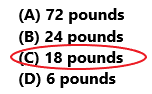The correct option is C.
Explanation:
The caterer wants to have enough turkey to feed 24 people. He wants to provide 3/4 of a pound of turkey for each person. Multiply 3/4 with 24 the product is 18 pounds. He need 18 pounds of turkey. So draw a circle to option C.

H.O.T. Algebra Find the unknown digit.

Question 6.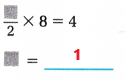The unknown digit is 1.
Explanation:
In the above image we can observe that numerator digit is missing. If we place 1 in the numerator then the product is 4. Multiply 1/2 with 8 the product is 4. So, the unknown digit is 1.

Question 7.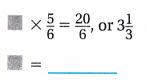The unknown digit is 4.
Explanation:
In the above image we can observe that one digit is missing. If we place 4 then the product is 20/6. Multiply 4 with 5/6 the product is 20/6 or 3(1/3). So, the unknown digit is 4.

Question 8.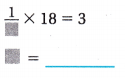The unknown digit is 6.
Explanation:
In the above image we can observe that denominator digit is missing. If we place 6 in the denominator then the product is 3. Multiply 1/6 with 18 the product is 3. So, the unknown digit is 6.

Question 9.
H.O.T. Multi-Step Patty wants to run $$\frac{5}{6}$$ of a mile every day for 5 days. Keisha wants to run $$\frac{3}{4}$$ of a mile every day for 6 days. Who will run the greater distance?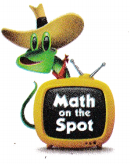Patty:
5 x (5/6) = 25/6 = 4(1/6)
Patty runs 4(1/6).
Keisha:
6 x (3/4) = 18/4 = 4(1/2)
Keisha runs 4(1/2).
Keisha runs the greater distance.
Explanation:
Patty wants to run 5/6 of a mile every day for 5 days. Multiply 5 with 5/6 the product is 25/6. The mixed fraction of 25/6 is 4(1/6). Patty runs 4(1/6). Keisha wants to run 3/4 a mile every day for 6 days. Multiply 6 with 3/4 the product is 18/4. The mixed fraction of 18/4 is 4(1/2). Keisha runs 4(1/2). Keisha runs the greater distance.

Question 10.
A heavy-duty snowmaking machine makes $$\frac{3}{4}$$ inch of snow each minute. How many inches of snow can the machine make in 8 minutes?
(A) 8 inches
(B) 6 inches
(C) 7$$\frac{1}{4}$$ inches
(D) 4$$\frac{1}{2}$$ inches(3/4) x 8 = 6 inches
The machine can make 6 inches of snow in 8 minutes.
So, option B is correct.
Explanation:
A heavy-duty snowmaking machine makes 3/4 inch of snow each minute. Multiply 8 minutes with 3/4 inch of snow the product is 6 inches. The machine can make 6 inches of snow in 8 minutes. So, option B is correct.

Question 11.
Connect Which has the same product as $$\frac{2}{3}$$ × 8?
(A) $$\frac{5}{6}$$ × 7
(B) $$\frac{1}{4}$$ × 13
(C) $$\frac{3}{8}$$ × 2
(D) $$\frac{1}{3}$$ × 16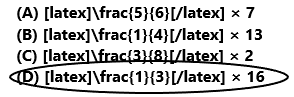(2/3) x 8 = 16/3
So, option D is correct.
Explanation:
The given expression (2/3) x 8. Multiply 2 with 8 the product is 16. The fraction is 16/3. So, option D is correct.

Question 12.
Multi-Step A baker made 5 pounds of icing. He used $$\frac{4}{9}$$ of the icing to decorate cakes. How much of the icing is left over?
(A) 1 pound
(B) 1$$\frac{5}{9}$$ pounds
(C) 1$$\frac{2}{3}$$ pounds
(D) 2$$\frac{7}{9}$$ pounds(4/9) x 5 = 20/9
He used 20/9 of the icing to decorate cakes.
5 – (20/9) = (45 – 20)/9 = 25/9 = 2(7/9)
2(7/9) of the icing is left over.
So, option D is correct.
Explanation:
A baker made 5 pounds of icing. He used 4/9 of the icing to decorate cakes. Multiply 4/9 with 5 the product is 20/9. He used 20/9 of the icing to decorate cakes. Subtract 20/9 from 5 the difference is 25/9. The mixed fraction of 25/9 is 2(7/9). The icing left over is 2(7/9). So, draw a circle for option D.

Texas Test Prep

Question 13.
Doug has 33 feet of rope. He wants to use $$\frac{2}{3}$$ of it for his canoe. How many feet of rope will he use for his canoe?
(A) 66 feet
(B) 22 feet
(C) 33 feet
(D) 11 feet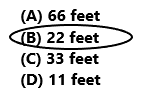(2/3) x 33 = 22 feet
He used 22 feet of rope for his canoe.
So, option B is correct.
Explanation:
Doug has 33 feet of rope. He wants to use 2/3 of it for his canoe. Multiply (2/3) with 33 the product is 22 feet. He used 22 feet of rope for his canoe. So, option B is correct.

### Texas Go Math Grade 5 Lesson 6.3 Homework and Practice Answer Key

Find the product. Write the product in the simplest form.

Question 1.
$$\frac{3}{7}$$ × 4 = ____________
(3/7) x 4 = (3 x 4)/7 = 12/7
The product is 12/7.
The simplest form of 12/7 is 1(5/7).
Explanation:
In the above image we can observe the expression (3/7) x 4. First multiply the two whole numbers in the numerator. Multiply 3 with 4 the product is 12. Write the product 12 over the denominator 7. Write the fraction 12/7 in simplest form as a mixed number. The mixed number is 1(5/7).

Question 2.
$$\frac{3}{5}$$ × 5 = ____________
(3/5) x 5 = (3 x 5)/5 = 15/5
The product is 15/5.
The simplest form of 15/5 is 3.
Explanation:
In the above image we can observe the expression (3/5) x 5. First multiply the two whole numbers in the numerator. Multiply 3 with 5 the product is 15. Write the product 15 over the denominator 5. Write the fraction 15/5 in simplest form as 3.

Question 3.
$$\frac{2}{3}$$ × 8 = ____________
(2/3) x 8 = (2 x 8)/3 = 16/3
The product is 16/3.
The simplest form of 16/3 is 5(1/3).
Explanation:
In the above image we can observe the expression (2/3) x 8. First multiply the two whole numbers in the numerator. Multiply 2 with 8 the product is 16. Write the product 16 over the denominator 3. Write the fraction 16/3 in simplest form as a mixed number. The mixed number is 5(1/3).

Question 4.
16 × $$\frac{3}{4}$$ = ____________
16 x (3/4) = (16 x 3)/4 = 48/4
The product is 48/4.
The simplest form of 48/4 is 12.
Explanation:
In the above image we can observe the expression 16 x (3/4). First multiply the two whole numbers in the numerator. Multiply 16 with 3 the product is 48. Write the product 48 over the denominator 4. Write the fraction 48/4 in simplest form as a 12.

Question 5.
9 × $$\frac{5}{6}$$ = ____________
9 x (5/6) = (9 x 5)/6 = 45/6
The product is 45/6.
The simplest form of 45/6 is 7(3/6).
Explanation:
In the above image we can observe the expression 9 x (5/6). First multiply the two whole numbers in the numerator. Multiply 9 with 5 the product is 45. Write the product 45 over the denominator 6. Write the fraction 45/6 in simplest form as a mixed number. The mixed number is 7(3/6).

Question 6.
6 × $$\frac{3}{8}$$ = ____________
6 x (3/8) = (6 x 3)/8 = 18/8 = 9/4
The product is 9/4.
The simplest form of 9/4 is 2(1/4).
Explanation:
In the above image we can observe the expression 6 x (3/8). First multiply the two whole numbers in the numerator. Multiply 6 with 3 the product is 18. Write the product 18 over the denominator 8. Write the fraction 9/4 in simplest form as a mixed number. The mixed number is 2(1/4).

Question 7.
$$\frac{2}{9}$$ × 5 = ____________
(2/9) x 5 = (2 x 5)/9 = 10/9
The product is 10/9.
The simplest form of 10/9 is 1(1/9).
Explanation:
In the above image we can observe the expression (2/9) x 5. First multiply the two whole numbers in the numerator. Multiply 2 with 5 the product is 10. Write the product 10 over the denominator 9. Write the fraction 10/9 in simplest form as a mixed number. The mixed number is 1(1/9).

Question 8.
$$\frac{4}{7}$$ × 3 = ____________
(4/7) x 3 = (4 x 3)/7 = 12/7
The product is 12/7.
The simplest form of 12/7 is 1(5/7).
Explanation:
In the above image we can observe the expression (4/7) x 3. First multiply the two whole numbers in the numerator. Multiply 4 with 3 the product is 12. Write the product 12 over the denominator 7. Write the fraction 12/7 in simplest form as a mixed number. The mixed number is 1(5/7).

Question 9.
$$\frac{3}{10}$$ × 7 = ____________
(3/10) x 7 = (3 x 7)/10 = 21/10
The product is 21/10.
The simplest form of 21/10 is 2(1/10).
Explanation:
In the above image we can observe the expression (3/10) x 7. First multiply the two whole numbers in the numerator. Multiply 3 with 7 the product is 21. Write the product 21 over the denominator 10. Write the fraction 21/10 in simplest form as a mixed number. The mixed number is 2(1/10).

Find the unknown digit.

Question 10.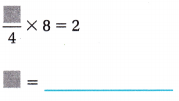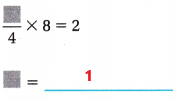The unknown digit is 1.
Explanation:
In the above image we can observe that numerator digit is missing. If we place 1 in the numerator then the product is 2. Multiply 1/4 with 8 the product is 2. So, the unknown digit is 1.

Question 11.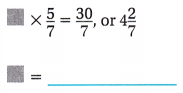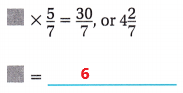The unknown digit is 6.
Explanation:
In the above image we can observe that one digit is missing. If we place 6 then the product is 30/7. Multiply 6 with 5/7 the product is 30/7 or 4(2/7). So, the unknown digit is 6.

Question 12.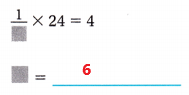The unknown digit is 6.
Explanation:
In the above image we can observe that denominator digit is missing. If we place 6 in the denominator then the product is 4. Multiply 1/6 with 24 the product is 4. So, the unknown digit is 6.

Question 13.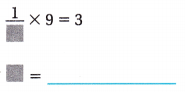The unknown digit is 3.
Explanation:
In the above image we can observe that denominator digit is missing. If we place 3 in the denominator then the product is 3. Multiply 1/3 with 9 the product is 3. So, the unknown digit is 3.

Question 14.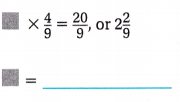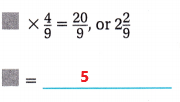The unknown digit is 5.
Explanation:
In the above image we can observe that one digit is missing. If we place 5 then the product is 20/9. Multiply 5 with 4/9 the product is 20/9. So, the unknown digit is 5.

Question 15.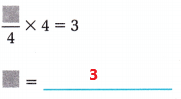The unknown digit is 3.
Explanation:
In the above image we can observe that numerator digit is missing. If we place 3 in the numerator then the product is 3. Multiply 3/4 with 4 the product is 3. So, the unknown digit is 3.

Problem Solving

Question 16.
Sandra exercised $$\frac{2}{3}$$ hour every day for two weeks while she was on vacation. How many hours did Sandra exercise during her vacation?
(2/3) x 14 = 28/3
Sandra exercise 28/3 hours during her vacation.
Explanation:
Sandra exercised 2/3 hour every day for two weeks while she was on vacation. In one week there are 7 days. Multiply 2/3 with 14 days the product is 28/3 hours. Sandra exercise 28/3 hours during her vacation.

Question 17.
Mike bought 15 baseball cards. Rookie players are featured on $$\frac{3}{5}$$ of the cards. How many cards feature rookie players?
15 x (3/5) = 9
The rookie players featured 9 cards.
Explanation:
Mike bought 15 baseball cards. Rookie players are featured on 3/5 of the cards. Multiply 15 with 3/5the product is 9. The rookie players featured 9 cards.

Lesson Check

Question 18.
The florist arranges a bouquet with 12 flowers. He decides that $$\frac{3}{4}$$ of the flowers in the bouquet will be carnations. How many carnations will the florist need to complete the bouquet?
(A) 10
(B) 0
(C) 6
(D) 3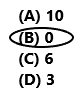12 x (3/4) = 9
In the bouquet 9 flowers are the carnations.
The florist need 0 flowers to complete the bouquet.
So, option B is correct.
Explanation:
The florist arranges a bouquet with 12 flowers. He decides that 3/4 of the flowers in the bouquet will be carnations. Multiply 12 with 3/4 the product is 9. In the bouquet 9 flowers are the carnations. The florist need 0 flowers to complete the bouquet. So, draw a circle to option B.

Question 19.
The average rainfall for each week for the last 4 weeks was $$\frac{7}{12}$$ inch. How much rain fell during the last 4 weeks?
(A) 2$$\frac{1}{3}$$ inches
(B) 4$$\frac{1}{12}$$ inches
(C) 2 inches
(D) $$\frac{11}{12}$$ inch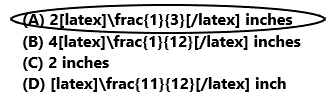4 x (7/12) = 7/3 = 2(1/3)
The rain fell during the last 4 weeks 2(1/3) inches.
So, option A is correct.
Explanation:
The average rainfall for each week for the last 4 weeks was 7/12 inch. Multiply 4 with 7/3 the product is 7/3. The fraction form 7/3 in mixed fraction is 2(1/3). The rain fell during the last 4 weeks 2(1/3) inches. So, draw a circle to option A.

Question 20.
Eric practiced for his piano recital $$\frac{3}{4}$$ hour every day last week. How many hours did Eric practice last week?
(A) 3$$\frac{3}{4}$$ hours
(B) 7 hours
(C) 5$$\frac{1}{4}$$ hours
(D) 2$$\frac{1}{2}$$ h0urs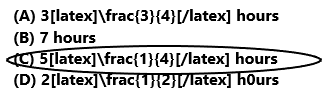7 x (3/4) = 21/4
Eric practiced 21/4 hours in last week.
So, option C is correct.
Explanation:
Eric practiced for his piano recital 3/4 hour every day last week. In one week there are 7 days. Multiply 7 with 3/4 the product is 21/4 hours. So, draw a circle to option C.

Question 21.
Which does not have the same product as 4 × $$\frac{5}{9}$$?
(A) 4 × $$\frac{9}{5}$$
(B) 5 × $$\frac{4}{9}$$
(C) 2 × $$\frac{10}{9}$$
(D) 10 × $$\frac{2}{9}$$4 x (9/5) = 36/5
So, option A is correct.
Explanation:
The product for the expression 4 x (5/9) is 20/9. The expression that does not have the product 20/9 is 4 x (9/5). The product for 4 x (9/5) is 36/5. So, draw a circle to option A.

Question 22.
Multi-Step Rose bought a dozen eggs. She used $$\frac{2}{3}$$ of the eggs to make custard and $$\frac{1}{4}$$ of the eggs to make an omelet. How many eggs does Rose have left?
(A) 9
(B) 4
(C) 3
(D) 1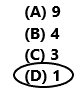(2/3) x 12 = 8
She used 8 of the eggs to make custard.
(1/4) x 12 = 3
She used 3 eggs to make an omelet.
8 + 3 = 11
12 – 11 = 1
So, option D is correct.
Explanation:
Rose bought a dozen eggs. She used 2/3 of the eggs to make custard. Multiply (2/3) with 12 the product is 8.  She used 8 of the eggs to make custard. She used 1/4 of the eggs to make an omelet. Multiply 1/4 with 12 the product is 3. Add 8 with 3 the sum is 11. Subtract 11 from 12 the difference is 1. So, draw a circle to option D.

Question 23.
Multi-Step Meredith’s class has 21 students. Meredith rides the bus home with $$\frac{2}{3}$$ of the students in her class. How many students in Meredith’s class do not ride the bus home with her?
(A) 14
(B) 16
(C) 7
(D) 6